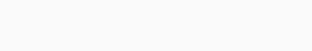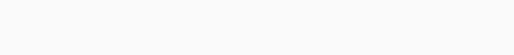# Selina Concise Mathematics Class 6 ICSE Solutions Chapter 29 The Circle - Learn Hool

## Selina Concise Mathematics Class 6 ICSE Solutions Chapter 29 The Circle

Selina Publishers Concise Mathematics Class 6 ICSE Solutions Chapter 29 The Circle

### The Circle Exercise 29A – Selina Concise Mathematics Class 6 ICSE Solutions

Question 1.
Use the figure given below to fill in the blanks :
(i) R is the …… of the circle.
(ii) Diameter of a circle is …… .
(iii) Tangent to a circle is … .
(iv) EF is a …… of the circle.
(v) …… is a chord of the circle.
(vi) Diameter = 2 x …..
(vii) ……. is a radius of the circle.
(viii) If the length of RS is 5 cm, the length of PQ = ……
(ix) If PQ is 8 cm long, the length of RS =…..
(x) AB is a ….. of the circleSolution:Question 2.
Draw a circle of radius 4.2 cm. Mark its centre as O. Take a point A on the circumference of the circle. Join AO and extend it till it meets point B on the circumference of the circle,
(i) Measure the length of AB.
(ii) Assign a special name to AB.
Solution:Question 3.
Draw circle with diameter :
(i) 6 cm
(ii) 8.4 cm.
In each case, measure the length of the radius of the circle drawn.
Solution:Question 4.
Draw a circle of radius 6 cm. In the circle, draw a chord AB = 6 cm.
(i) If O is the centre of the circle, join OA and OB.
(ii) Assign a special name to ∆AOB
(iii) Write the measure of angle AOB.
Solution:Question 5.
Draw a circle of radius 4.8 cm and mark its centre as P.
(i) Draw radii PA and PB such that ∠APB = 45°.
(ii) Shade the major sector of the circle
Solution:Question 6.
Draw a circle of radius 3.6 cm. In the circle, draw a chord AB = 5 cm. Now shade the minor segment of the circle.
Solution:Question 7.
Mark two points A and B ,4cm a part, Draw a circle passing through B and with A as a centerSolution:Question 8.
Draw a line AB = 8.4 cm. Now draw a circle with AB as diameter. Mark a point C on the circumference of the circle. Measure angle ACB.
Solution:### The Circle Exercise 29B – Selina Concise Mathematics Class 6 ICSE Solutions

Question 1.
Construct a triangle ABC with AB = 4.2 cm, BC = 6 cm and AC = 5cm. Construct the circumcircle of the triangle drawn.
Solution:Question 2.
Construct a triangle PQR with QR = 5.5 cm, ∠Q = 60° and angle R = 45°.
Construct the circumcircle cif the triangle PQR.
Solution:Question 3.
Construct a triangle ABC with AB = 5 cm, ∠B = 60° and BC = 6. 4 cm.
Draw the incircle of the triangle ABC.
Solution:Question 4.
Construct a triangle XYZ in which XY = YZ= 4.5 cm and ZX = 5.4 cm. Draw the circumcircle of the triangle and measure its circumradius.
Solution:Question 5.
Construct a triangle PQR in which, PQ = QR = RP = 5.7 cm. Draw the incircle of the triangle and measure its radius.
Solution:### The Circle Revision Exercise – Selina Concise Mathematics Class 6 ICSE Solutions

Question 1.
The centre of a circle is at point O and its radius is 8 cm. State the position of a point P (point P may lie inside the circle, on the circumference of the circle, or outside the circle), when :
(a) OP = 10.6 cm
(b) OP = 6.8 cm
(c) OP = 8 cm
Solution:Question 2.
The diameter of a circle is 12.6 cm. State, the length of its radius.
Solution:Question 3.
Can the length of a chord of a circle be greater than its diameter ? Explain.
Solution:Question 4.
Draw a circle of diameter 7 cm. Draw two radii of this circle such that the angle between these radii is 90°. Shade the minor sector obtained. Write a special name for this sector.
Solution:Question 5.
State, which of following statements are true and which are false :
(i) If the end points A and B of the line segment lie on the circumference of a circle, AB is a diameter.
(ii) The longest chord of a circle is its diameter.
(iii) Every diameter bisects a circle and each part of the circle so obtained is a semi-circle.
(iv) The diameters of a circle always pass through the same point in the circle.
Solution:#### Selina Concise Mathematics Class 6 ICSE Solutions

@ Designed By : Vikas Copyright 2023 @ LearnHool.In